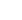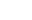(972) 865-6205info@privatecapitalinvestors.com

# What is Yield on Cost in CRE Development?

There are a lot of metrics used by developers and investors to evaluate the worth of a commercial real estate (CRE) property.

Out of these, yield on cost is one of the most-used metrics that allows developers to verify whether the investment risk is worth it or not. It is also known as the development yield and allows developers to precisely measure the returns and the involved risks.

Interested to know more about what yield on cost in CRE development means and how it can help developers and investors get better deals? Get started with the article below and learn how yield on cost can help you better evaluate commercial opportunities and deals.

## What Is The Yield On Cost?

The yield on cost is a metric that is widely used across the industry and allows CRE investors to calculate the return on investment on a real estate project. It is also used to calculate the development spread of a commercial property. But, what exactly does the term development spread mean?

Well, development spread is the difference between the market-based cap rate for similar, existing buildings in the same market and the yield on the cost of commercial real estate properties. It also lets developers and investors evaluate the additional return that you get in exchange for all the risk involved in the construction and development of a CRE project.

However, the term yield on cost is referred to by different terms like the going-in cap rate, development yield, cost cap rate, return on cost, and build-to rate. However, the term used depends on the context of the commercial real estate project that investors or developers are looking at!

## What Is The Formula For Calculating Yield On Cost In CRE?

In mathematical terms, the yield on cost in commercial real estate is the ratio of a property’s projected stabilized Net Operating Income (NOI) and the projected total cost of the property.

Let us suppose that the projected stabilized net operating income of a project is \$100,000 and its total cost is \$1,000,000. Then, the Yield On the Cost of the project would be 1/10 or 10%.

## How Can You Calculate Yield On Cost?

To help you better understand the concept of yield on cost, let us look at some different examples. These practical examples will help you understand how to calculate the yield on cost for a development cost, a value-added project, and a construction or renovation project.

### Development Example Of Yield On Cost Calculation

In the following section, we will look at an example of a real estate development project and calculate its yield on cost.

Let us suppose that a piece of land is available for sale and you want to evaluate whether it would be financially beneficial to invest in building a small commercial building on it or not.

Now, based on your local market research and experience with previous projects, you calculate the developmental cost of the project which comes to approximately \$3,900,000. This cost includes approximately \$800,000 for land costs, \$2,600,000 for hard costs, and \$500,000 for soft costs.

Now, as for the building, the total rentable space is approximately 14,000 sq. ft. and as per your calculations, the expected stabilized vacancy rate comes to approximately 5%. According to the current industry standard, the market rate is \$28 per sq. ft. and you expect a payment of about \$10 per sq. ft. as fixed operating expenses.

As the landlord, you can expect the other operating expenses to be reimbursed by the tenants which is why we ignore them.

Based on these assumptions, our calculations are as follows:-

Potential Gross Income   \$392000
– Vacancy & Credit Loss   5%
= Effective Gross Income   \$372,400
– Operating Expenses   -\$140,000
= Net Operating Income   \$232,400

Now, you must also evaluate other similar projects that already exist in the area and are newly constructed. Let us assume that they are trading at a market cap rate of 6.5% and get ahead with our calculation.

Since the expected stabilized NOI is \$232,400 and the total project cost is approximately \$3,900,000, the unleveled yield on cost is 232,400/3,900,000, i.e. 5.9%. But, why is it said to be unleveled? Well, since we do not consider any commercial real estate debt financing, it is considered to be the unleveled yield on cost.

It is clear that the calculated yield on cost is lower than the latest market-based cap rate of similar projects.

This means that investing in a similar yet already existing building in the same market can yield you a 6% return which is higher than the calculated 5.9%. So, it is better not to bear any headache or developmental risk for a lower return rate.

### Value Add Example of Yield on Cost Calculation

In this section, we will take an example where we need to evaluate a small multifamily property that you’re planning to acquire.

Let us suppose that the acquisition cost of the property is about \$2,500,000 and you’re planning to vacate all tenants after. Your plan is to renovate the property with a total construction budget of \$750,000.

Then, you want to lease these property units at higher rents due to the added value and also plan to boost their operational efficiency.

According to your calculations, the renovations will increase the property’s stabilized net operating income to \$312,500. You also find out that similar renovated apartments in the area have a selling rate of 8% market cap rate.

Let’s calculate whether your project of acquiring and remodeling this property makes sense or not. So, the yield on cost once the renovations are done is calculated as follows:-

This clearly shows that the return is 1.6% higher than the cap rate of 8% of similar renovated projects which means that it is profitable. But, another question you must ask yourself is whether the 1.6% higher return rate justifies the burden and risk of project acquisition and renovation you need to take to take the project to its completion.

The answer to this is highly subjective and you must focus on getting an answer before you decide to invest in the project.

However, there is a method that can help you make a concrete decision in no time – convert the 1.6% spread to a profit margin percentage and then calculate the total dollar profit amount.

To do so, we take the 9.6% return, divide it by the market cap of 8%, and subtract 1 from the total value. So, (9.6/8)-1 gives 20%, which means that you can expect a profit margin of 20%. When we multiply this profit margin by the total cost of \$3,250,000, the total expected profit comes to about \$650,000

This value is the approximate return that you would make after you sell the building after completing the project and stabilizing it.

### Yield on Cost for Specific Value-Add Projects

A simple and easy way to calculate the yield on cost for specific renovation projects is to take into account the additional income generated after the project’s renovation and divide it by the renovation cost incurred.

For example, you might be thinking of investing \$100,000 into a renovation project and expect the NOI to increase by \$20,000 after the project is completed. This means that the yield on cost for this renovation project is 20,000/100,000, or 20%.

In case this yield on cost is greater than the yield on cost of the project before the renovation, the project makes sense. Otherwise, it does not create value and it might not be a good idea to invest in commercial real estate property.

## What Is The Relationship Between Yield On Cost And Development Spread?

One of the components used by developers and investors to calculate a project’s development spread is the project’s yield on cost. The development spread is a metric that is used to calculate the difference between the yield on cost and the market-based cap rate of similar projects that are already existing and stabilized for sale in the same market.

Let us suppose that the yield on cost for a potential commercial real estate project is estimated to be 9% while the average market cap for similar and already stabilized properties is 7%. Then, the development spread would be (9-7)% = 2%.

This development spread value tells us how much the additional return would be when you choose to develop a new project instead of choosing to invest in an existing project that is already stabilized and in the same market.

We can also use it to accurately measure the profit margin of the project by dividing the yield on cost by the market cap rate and subtracting 1. In our example, the profit margin would be = (9/7) – 1 = 28.5%.

## What Is The Difference Between The Metrics Yield On Cost And Cap Rate?

Now that we have an idea about what yield on cost means, let us understand how it is different from the cap rate.

Well, the cap rate is the ratio of a property’s net operating income to its market value. Although the yield on cost is similar, it uses the total cost in the equation’s denominator instead of the property’s market value. Since the yield on cost and cap rate are used to measure different things, they are often calculated together and compared to each other.

## What Are The Limitations Of Yield On Cost?

The main issue with the yield on cost is that it gives us a measure of the return for a single year and does not consider the other years in which you hold the property. In some cases, this might mean functioning on the basis of incomplete data and can lead to several issues.

It is always better to create a multi-year proforma to gain better insight into what you can expect over the entire period in which you hold the property.

This will ultimately prepare you for the multiple possible outcomes and situations you might face to help you easily determine the best, worst, and most likely scenarios. Although it can be difficult to create a multi-year analysis, you can get in touch with our experts and get one created efficiently.

## Conclusion

In the above article, we looked at yield on cost and how it is an important metric that must be considered by commercial real estate investors and developers. We hope we were able to break down the different factors at play to help you better analyze your investment opportunities.

Looking for expert help to ensure you make better investment decisions in commercial real estate? Get in touch with us to get started on your journey to better investment deals and never look back again!

## Why Commercial Real Estate is in Big Trouble?

Real estate investments, no doubt, are quite attractive. In fact, the availability of the wide number of choices here makes it the perfect option for passive income over a longer period. However, out of all, commercial real estate is highly in demand, but you must...

## How to Refinance Your Commercial Property in Market Slow Down?

The commercial real estate industry investment can be quite complicated. There are a lot of things one needs to know, especially when it comes to refinancing. Whether you are a new investor or you are considering refinancing, you can find all the details mentioned...

## An Overview of Hard Money Loan Interest Rate

Hard money loans are the savior for those who are unable to acquire financing through traditional means. However, you must know the interest rate of the type of hard money loans can be much higher when compared to those during 2020-22. Now, the interest rate of...